# Mitscherlich-type response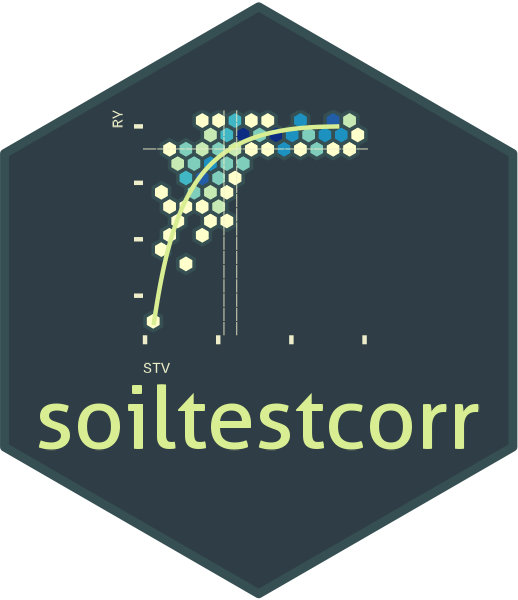## Description

This tutorial demonstrates the mitscherlich() function for fitting a continuous response model and estimating a critical soil test value. This function fits a Mitscherlich-type exponential regression model that follows a diminishing growth curve, and is sometimes also referred to as exponential “rise-to-the-max”. Cerrato and Blackmer (1990) expressed it as:

$y = a * (1-e^{-c(x + b)})$

where
a = asymptote,
b = model-fitting parameter ( $$-b$$ = X-intercept),
c = curvature parameter.

This exponential model is extensively used in agriculture to describe crops response to input since the biological meaning of its curved response. The mitscherlich() function works automatically with self-starting initial values to facilitate the model’s convergence. The mitscherlich() function allows the user to control the number of parameters, effectively constraining the response curve if theoretically justified:

1. type = 1, "no restriction", or "free" (DEFAULT): three parameter model; $$y = a * (1-e^{-c(x + b)})$$
2. type = 2, "asymptote 100", or "100": two parameter model where asymptote = 100% RY; $$y = 100 * (1-e^{-c(x + b)})$$
3. type = 3, "asymptote 100 from 0", or "fixed": one parameter model in which only the curvature varies and asymptote = 100 and model goes through origin; $$y = 100 * (1-e^{-cx})$$.

• lacks a parameter that can be directly interpreted as the critical soil test value

• the model cannot be evaluated at the asymptote as CSTV would go to Inf

• a fixed RY target for CSTV may be a somewhat arbitrary choice, but 95% is commonly used

• model may not reach 95%, for which NaN results

• there is no apparent confidence interval for the derived CSTV. For this latter purpose, we recommend the user to use the boot_mitscherlich() function for a reliable confidence interval estimation of parameters and CSTV via bootstrapping (resampling with replacement).

## General Instructions

1. Load your dataframe with soil test value (stv) and relative yield (ry) data.
2. Specify the following arguments into the function mitscherlich():
1. type select the type of parameterization of the model (type = 1, 2, or 3; see above)
2. data (optional)
3. stv (soil test value) and ry (relative yield) columns or vectors,
4. target (default = 95) to calculate the STV at a specific ry target.
5. tidy TRUE-default- (produces a data.frame with results) or FALSE (store results as list)
6. plot TRUE (produces a ggplot as main output) or FALSE (no plot, only results as data.frame)
7. resid TRUE (produces plots with residuals analysis) or FALSE (no plot)
3. Run and check results.
4. Check residuals plot, and warnings related to potential limitations of this model.
5. Adjust curve plots as desired.

# Tutorial

library(soiltestcorr)

Suggested packages

# Install if needed
library(ggplot2) # Plots
library(dplyr) # Data wrangling
library(tidyr) # Data wrangling
library(purrr) # Mapping

# Native fake dataset from soiltestcorr package
corr_df <- soiltestcorr::data_test

# Fit mitscherlich()

## 1. Individual fits

### 1.1. Different number of parameters type = #


# Type = 1, no restriction (3 parameters)
mitscherlich(corr_df, STV, RY, type = 1)
#> # A tibble: 1 × 13
#>   asymptote     b curvature equat…¹ y_int…² target  CSTV   AIC  AICc   BIC    R2
#>       <dbl> <dbl>     <dbl> <chr>     <dbl>  <dbl> <dbl> <dbl> <dbl> <dbl> <dbl>
#> 1      98.0  3.91    0.0885 98(1-e…    28.6     95  35.5 1022. 1022. 1033.  0.54
#> # … with 2 more variables: RMSE <dbl>, pvalue <dbl>, and abbreviated variable
#> #   names ¹​equation, ²​y_intercept
# Type = 2, fixed asymptote value at 100 (2 parameters)
mitscherlich(corr_df, STV, RY, type = 2)
#> # A tibble: 1 × 13
#>   asymptote     b curvature equat…¹ y_int…² target  CSTV   AIC  AICc   BIC    R2
#>       <dbl> <dbl>     <dbl> <chr>     <dbl>  <dbl> <dbl> <dbl> <dbl> <dbl> <dbl>
#> 1       100  5.49    0.0763 100(1-…    34.2     95  33.8 1021. 1021. 1029.  0.53
#> # … with 2 more variables: RMSE <dbl>, pvalue <dbl>, and abbreviated variable
#> #   names ¹​equation, ²​y_intercept
# Type = 3, fixed origin at 0 and asymptote at 100 (1 parameters)
mitscherlich(corr_df, STV, RY, type = 3)
#> # A tibble: 1 × 13
#>   asymptote     b curvature equat…¹ y_int…² target  CSTV   AIC  AICc   BIC    R2
#>       <dbl> <dbl>     <dbl> <chr>     <dbl>  <dbl> <dbl> <dbl> <dbl> <dbl> <dbl>
#> 1       100     0     0.108 100(1-…       0     95  27.8 1031. 1032. 1037.  0.49
#> # … with 2 more variables: RMSE <dbl>, pvalue <dbl>, and abbreviated variable
#> #   names ¹​equation, ²​y_intercept

### 1.2. tidy = FALSE

It returns a LIST (may more efficient for multiple fits at once)


# Using dataframe argument, tidy = FALSE -> return a LIST
mitscherlich(data = corr_df, STV, RY, target = 90,  tidy = FALSE)
#> $asymptote #>  97.98376 #> #>$b
#>  3.907871
#>
#> $curvature #>  0.08850352 #> #>$equation
#>  "98(1-e^(-0.089(x+3.9))"
#>
#> $y_intercept #>  28.64934 #> #>$target
#>  90
#>
#> $CSTV #>  24.42311 #> #>$AIC
#>  1021.64
#>
#> $AICc #>  1021.94 #> #>$BIC
#>  1033.32
#>
#> $R2 #>  0.54 #> #>$RMSE
#>  9.78
#>
#> $pvalue #>  0 ### 1.3. Alternative using the data frame vectors You can call stv and ry vectors using the $. The tidy argument still applies for controlling the output type.


fit_vectors_list <-mitscherlich(stv = corr_df$STV, ry = corr_df$RY,
tidy = FALSE)

fit_vectors_tidy <-mitscherlich(stv = corr_df$STV, ry = corr_df$RY,
tidy = TRUE)

## 2. Multiple fits at once

# Example 1. Fake dataset manually created
data_1 <- data.frame("RY"  = c(65,80,85,88,90,94,93,96,97,95,98,100,99,99,100),
"STV" = c(1,2,3,4,5,6,7,8,9,10,11,12,13,14,15))

# Example 2. Native fake dataset from soiltestcorr package
data_2 <- soiltestcorr::data_test

# Example 3. Native dataset from soiltestcorr package, Freitas et al.  (1966), used by Cate & Nelson (1971)
data_3 <- soiltestcorr::freitas1966 %>%
rename(STV = STK)

data.all <- bind_rows(data_1, data_2, data_3, .id = "id")

Note: the stv column needs to have the same name for all datasets if binding rows.

### 2.1. Using map()


# Run multiple examples at once with map()
data.all %>%
nest(data = c("STV", "RY")) %>%
mutate(model = map(data, ~ mitscherlich(stv = .$STV, ry = .$RY))) %>%
unnest(model)
#> # A tibble: 3 × 15
#>   id    data     asymptote     b curvature equation y_int…¹ target   CSTV    AIC
#>   <chr> <list>       <dbl> <dbl>     <dbl> <chr>      <dbl>  <dbl>  <dbl>  <dbl>
#> 1 1     <tibble>      98.7  2.07    0.371  98.7(1-…    52.8     95   6.77   64.0
#> 2 2     <tibble>      98.0  3.91    0.0885 98(1-e^…    28.6     95  35.5  1022.
#> 3 3     <tibble>      96.4 -8.69    0.0458 96.4(1-…   -47.2     95 101.    187.
#> # … with 5 more variables: AICc <dbl>, BIC <dbl>, R2 <dbl>, RMSE <dbl>,
#> #   pvalue <dbl>, and abbreviated variable name ¹​y_intercept

### 2.2. Using group_modify()

Alternatively, with group_modify(), nested data is not required. However, it still requires a grouping variable (in this case, id) to identify each dataset. group_map() may also be used, though list_rbind() is required to return a tidy data frame of the model results instead of a list.


data.all %>%
group_by(id) %>%
group_modify(~ soiltestcorr::mitscherlich(data = ., STV, RY))
#> # A tibble: 3 × 14
#> # Groups:   id 
#>   id    asymp…¹     b curva…² equat…³ y_int…⁴ target   CSTV    AIC   AICc    BIC
#>   <chr>   <dbl> <dbl>   <dbl> <chr>     <dbl>  <dbl>  <dbl>  <dbl>  <dbl>  <dbl>
#> 1 1        98.7  2.07  0.371  98.7(1…    52.8     95   6.77   64.0   68.0   66.8
#> 2 2        98.0  3.91  0.0885 98(1-e…    28.6     95  35.5  1022.  1022.  1033.
#> 3 3        96.4 -8.69  0.0458 96.4(1…   -47.2     95 101.    187.   189.   192.
#> # … with 3 more variables: R2 <dbl>, RMSE <dbl>, pvalue <dbl>, and abbreviated
#> #   variable names ¹​asymptote, ²​curvature, ³​equation, ⁴​y_intercept

## 3. Bootstrapping

A suitable alternative for obtaining confidence intervals for parameters or derived quantities is bootstrapping. Bootstrapping is a resampling technique (with replacement) that draws samples from the original data with the same size. If you have groups within your data, you can specify grouping variables as arguments in order to maintain, within each resample, the same proportion of observations than in the original dataset.

This function returns a table with as many rows as the resampling size (n) containing the results for each resample.

set.seed(123)
boot_mits <- boot_mitscherlich(corr_df, STV, RY, target = 90, n = 200)
#> Warning: There were 2 warnings in dplyr::mutate().
#> The first warning was:
#> ℹ In argument: model = map(...).
#> ℹ In group 51: boot_id = 51.
#> Caused by warning in nls.lm():
#> ! lmdif: info = -1. Number of iterations has reached maxiter' == 50.
#> ℹ Run dplyr::last_dplyr_warnings() to see the 1 remaining warning.

#> # A tibble: 5 × 13
#>   boot_id asymptote     b curvature y_int…¹ target  CSTV   AIC  AICc   BIC    R2
#>     <dbl>     <dbl> <dbl>     <dbl>   <dbl>  <dbl> <dbl> <dbl> <dbl> <dbl> <dbl>
#> 1       1      98.8  2.79    0.0856    21.0     90  25.4 1029. 1029. 1041.  0.55
#> 2       2      99.0  8.28    0.0682    42.7     90  26.9 1021. 1021. 1033.  0.47
#> 3       3      98.8 10.8     0.0660    50.4     90  25.9 1007. 1008. 1019.  0.42
#> 4       4      98.9  7.01    0.0772    41.3     90  24.2  989.  989. 1001.  0.57
#> 5       5     100.  11.0     0.0630    50.3     90  24.9  986.  986.  997.  0.48
#> # … with 2 more variables: RMSE <dbl>, pvalue <dbl>, and abbreviated variable
#> #   name ¹​y_intercept

# CSTV Confidence Interval
quantile(boot_mits\$CSTV, probs = c(0.025, 0.5, 0.975), na.rm = TRUE)
#>     2.5%      50%    97.5%
#> 20.22419 24.60084 28.75092

# Plot
boot_mits %>%
ggplot2::ggplot(aes(x = CSTV))+
geom_histogram(color = "grey25", fill = "#9de0bf", bins = 10)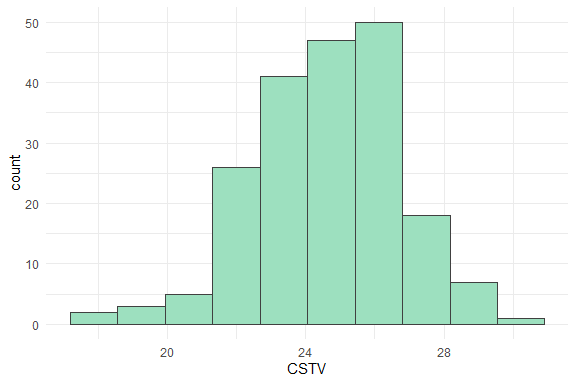## 4. Plots

### 4.1. Calibration Curve

We can generate a ggplot with the same mitscherlich() function.

We just need to specify the argument plot = TRUE.

data_3 <- soiltestcorr::freitas1966

plot_mit <- mitscherlich(data_3, STK, RY, plot = TRUE)

plot_mit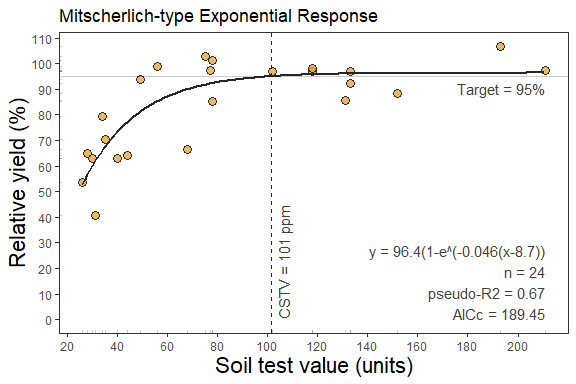### 4.2 Fine-tune the plots

As ggplot object, plots can be adjusted in several ways, such as modifying titles and axis scales.

plot_mit +
# Main title
ggtitle("My own plot title")+
# Axis titles
labs(x = "Soil Test K (ppm)",
y = "Cotton RY(%)") +
# Axis scales
scale_x_continuous(limits = c(20,220),
breaks = seq(0,220, by = 10))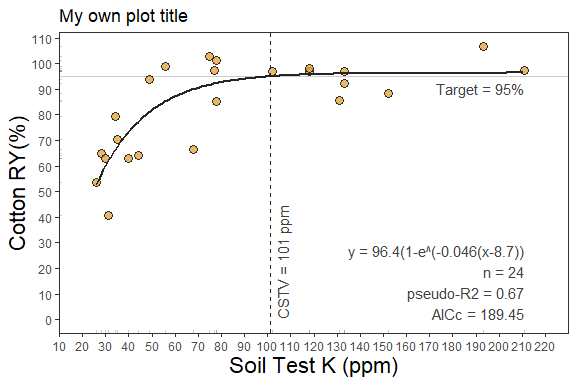### 4.3. Residuals

Set the argument resid = TRUE.


# Residuals plot
mitscherlich(data_3, STK, RY, resid = TRUE)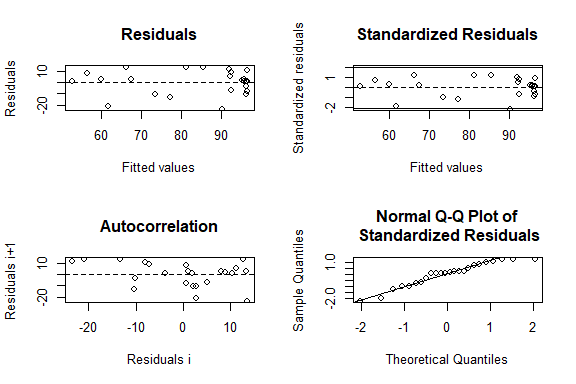#> # A tibble: 1 × 13
#>   asymptote     b curvature equat…¹ y_int…² target  CSTV   AIC  AICc   BIC    R2
#>       <dbl> <dbl>     <dbl> <chr>     <dbl>  <dbl> <dbl> <dbl> <dbl> <dbl> <dbl>
#> 1      96.4 -8.69    0.0458 96.4(1…   -47.2     95  101.  187.  189.  192.  0.67
#> # … with 2 more variables: RMSE <dbl>, pvalue <dbl>, and abbreviated variable
#> #   names ¹​equation, ²​y_intercept`